Courses

# Reinforced Concrete Thin Shell Structures Civil Engineering (CE) Notes | EduRev

## Civil Engineering (CE) : Reinforced Concrete Thin Shell Structures Civil Engineering (CE) Notes | EduRev

The document Reinforced Concrete Thin Shell Structures Civil Engineering (CE) Notes | EduRev is a part of Civil Engineering (CE) category.
All you need of Civil Engineering (CE) at this link: Civil Engineering (CE)

Reinforced Concrete Thin Shell Structures
» Thin shell
o Definition – A thin shell is a curved slab whose thickness h is small compared with its other dimensions and compared with its principal radius of curvature.
o Middle surface The surface that bisects the shell is called the middle surface. It specifies the form of this surface and the thickness h at every point.
o Analysis of thin shells consists the following steps:
• Establish equilibrium of a differential element cut from the shell
• Achieve strain compatibility so that each element remains continuous with each adjacent element after deformation.
o Stress resultants and stress couples

» Shell theories
o The Kirchhoff-Love theory – The first-approximation of shells
• Assumptions:
(1) The shell thickness is negligibly small in comparison with the least radius of curvature of the shell middle surface.
(2) Strains and displacements that arise within the shells are small.
(3) Straight lines that are normal to the middle surface prior to deformation remain straight and normal to the middle surface during deformation, and experience no change in length.
(Analogous to Navier’s hypothesis for beams – Bernoulli-Euler theory for beams)
(4) The direct stress acting in the direction normal to the shell middle surface is negligible.

• Results of the assumptions:
– Normal directions to the reference surface remain straight and normal to the deformed reference surface.
– The hypothesis precludes any transverse-shear strain, i.e., no change in the right angle between the normal and any line in the surface.
– It is strictly applicable to thin shells.
– It is not descriptive of the behavior near localized loads or junctions. (Assumption (4) is not valid in the vicinity of concentrated transverse loads.)

o The Flügge-Byrne theory – The second-approximation of shells
• Assumptions:
– It adopts only assumption (2).
– It is referred to as “higher-order approximations” of the Kirchhoff-Love assumptions

» Classification of shells:
o Classified by governing equation of geometry:
• Paraboloid of revolution
• Hyperboloid of revolution
• Circular cylinder
• Elliptic paraboloid
• Hyperbolic paraboloid
• Circular cone

» Geometrical analysis of shells:
o Orthogonal curvilinear coordinates
Consider the position vector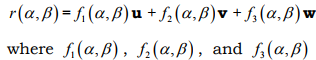are continuous, single-valued functions. The surface is determined by α and β uniquely. α and β are called curvilinear coordinates. u, v, and w are unit vectors in the Cartesian coordinate system.
Orthogonality is addressed as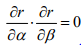→  Inner product is zero.

The distance between (α,β ) and (α + dα β, + dβ ) is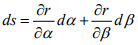The scalar product of ds with itself is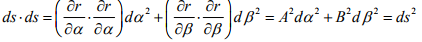where A and B are called Lame’s parameters or measure numbers. The formula shown above is the first quadratic form of the theory of surfaces.
o Principal radii of curvature
o Gaussian curvature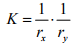where rx  and rare the principal radii of curvature.
– K > 0: Synclastic shells, i.e., spherical domes and elliptic paraboloids.
– K = 0 (either ror r is zero): Single-curvature shells, i.e., cylinders and cones.

– K < 0: Anticlastic shells, i.e., hyperbolic paraboloids and hyperbolas of revolution.

» Analysis of cylindrical shell:
o Discussion of behavior of singly curved vs. doubly curved shells
o Definition:
A cylindrical shell may be defined as a curved slab which has been cut form a full cylinder. The slab is bounded by two straight “longitudinal” edges parallel to the axis of the cylinder and by two curved transverse edges in planes perpendicular to the axis; the slab is curved in only one direction. The cylindrical shell is circular when the curvature is constant.
o Effects of shell edges on the load carrying behavior
o Stress resultants and stress couples of cylindrical shells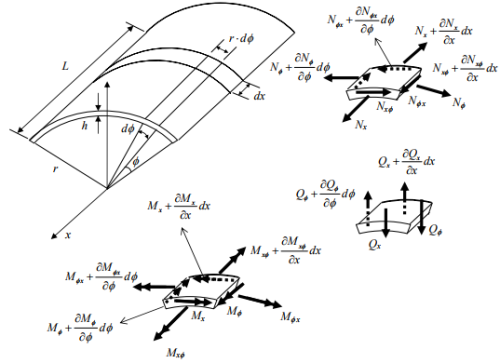• 10 unknowns: (Nx, Nφ, N, Nφx,), (Qx, Qφ ), (Mx, Mφ, Mxφ, Mφx).
→ The problem is statically indeterminate.
• In most R/C cylindrical shells, Mx will be small and Qx will be small.
→ Mxφ, Mφ x are negligible.
→ 6 unknowns (Nx, Nφ, N, Nφx), (Qφ, Mφ).

o Line loads: Forces applied along the free edge.
o Classification:
• Long shells →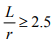Line loads produce significant magnitudes of Mφ and Qφ , membrane forces become insignificant. Stresses can be estimated using the beam theory.

• Intermediate shells →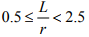• Short shells →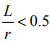The line loads produce internal forces generally in the region near the longitudinal edge. Greater part of the shell behaves with membrane values.

o Beam theory for long cylindrical shells
• For long shells the stresses can be estimated closely by beam theory. The shell is considered as a beam of a curved cross section between end supports.
• Assumption: Relative displacements within each transverse cross section are negligible.

o The extension of the beam method of analysis to multiple shells
o Membrane theory
– For a certain class of shells which the stress couples are an order of magnitude smaller than the extensional and in-plane shear stress resultants, the transverse shear stress resultants are similarly small and may be neglected in the force equilibrium.
– The assumption is valid only if at least one radius of curvature is finite. (Flat plates are excluded from resisting transverse loading in this manner.)
– The shell may achieve force equilibrium through the action of inplane forces alone. Hence, the state of stress in the shell is completely determined by equations of equilibrium, i.e., the shell is statically determinate.
– The boundary conditions must provide for those shell edge forces which are computed from the equations of equilibrium. The boundary conditions must also permit those shell edge displacements (translations and rotations) which are computed from the forces found by the membrane theory.

o Approximation methods of analysis
• Energy method
– Strain energy and potential energy
• Rayleigh-Ritz methods
• Galerkin method

» Nonlinear analysis using doubly-curved isoparametric thin shell R/C elements
o Assumptions:
• The actual reinforcing bars are represented by equivalent anisotropic steel layers by making appropriate adjustments to the elastic-plastic incremental strain-to-stress transformation matrix.
These layers carry uniaxial stress only in the same direction as the actual bars. Dowel action is neglected.
• Strain compatibility between steel and concrete is maintained.
o Constitutive law for isotropic materials In matrix form:
{σ} = [C]{ε}
o Stress resultant-strain, and stress couple-curvature relations
In matrix form: {N D } = [ ]{ε}
→ More complex material properties can be represented through a modification of [C] and [D].
o Incremental stress-strain relation
{d D σ} = [ ]{dε}
where {dσ} = increment of total stress vector,
[D] = the elastic-plastic incremental strain-to-stress transformation matrix, and
{dε} = increment of total strain vector.

o Generalized stress-strain relation for full concrete elements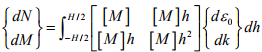where
dN = increment of direct stress resultant,
d= increment of bending stress resultant,
0 = mid-wall component of strain increment,
dk = bending component of the strain increment, and
H = the wall thickness.

o Numerical integration in finite element analysis
• At each integration point of the element the shell wall is divided into a number of stations with constant intervals, T, through the thickness.
• Using [D] , the matrix [M ] is evaluated at the start of each increment and for each of the stations.

o Incremental stress-strain relation for steel layer element with anisotropic properties consistent with uniaxial condition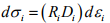where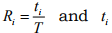= the portion of the equivalent steel area contributed to the i th integration station.

o The generalized stress-strain relation at station i: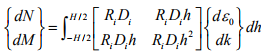For integration stations with no reinforcement contribution, the Ri multiplier will be zero.

» Design of cylindrical concrete shells
o ASCE method – Solution is based on superposition.
o Steps of the method:
1. Assume that the surface loads are transmitted to the supports by direct stress only. This is the membrane solution, equivalent to assuming the structures to be statically determinate. This assumption leads to displacements and reactions along the long edges which are inconsistent with the actual boundary condition.
→ Surface loads – The membrane analysis of a cylindrical shell subjected to surface loads involves only successive differentiations and interpolations of the radial and tangential components of the surface load. This is always possible for continuous loads. For most conditions the surface loads on the shell are uniform.
→ Representation of loads using Fourier series

2. Apply line loads along the long edges. The stresses from line loads are superimposed with those from membrane solution.
→ Line loads along the longitudinal edges
→ The effect of edge line loads on the shell

o Definitions
• The primary system is obtained by reducing the general theory to the membrane theory which reduces to three equations with three unknowns, and hence to a statically determinate system.
• The errors correspond to the incompatible edge effects.
• The corrections correspond to unit edge effects derived from a bending theory.
• Compatibility is obtained by determining the size of the corrections required to remove the errors in the membrane theory.

o Edge beams
• Vertical beams
– Usually employed for long shells, where the principal structural action is longitudinal bending.
• Horizontal beams
– Typically used with short shells, where the principal structural action is transverse arching.

o Edge effects
– K > 0: The edge effects tend to damp rapidly and are usually confined to a narrow zone at the edge. Thus in these shells the membrane theory will often be valid throughout the entire shell except just at the boundaries
– K = 0 (either r  or ris zero): The edge effects are damped out but tend to extend further into the shell than for shells of positive curvature.
– K < 0: The damping is markedly less than for the others. The boundary effects tend to become significant over large portions of the shell.

o Reinforcement patterns of a simply supported single shell
I: Longitudinal reinforcement in the edge beam
II: Transverse membrane and bending reinforcement
III: Shear reinforcement
IV: Negative moment bending reinforcement near the diaphragms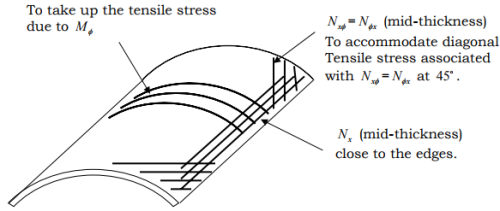» Design considerations
o Analysis is based on homogeneous isotropic material assumption. Principal stresses are computed from the values for the in-plane stress resultants, and stress trajectories are drawn; reinforcement is placed to carry tensile stresses.
o Transverse reinforcement must be provided to resist bending moment Mφ . Transverse shear Qφ and thrust Nφ are usually small and do not influence design.

o Other Design Issues
• Boundary conditions; edge beam compatibility and design
• Instability – Buckling
• Minimum reinforcement requirements

Offer running on EduRev: Apply code STAYHOME200 to get INR 200 off on our premium plan EduRev Infinity!

,

,

,

,

,

,

,

,

,

,

,

,

,

,

,

,

,

,

,

,

,

;